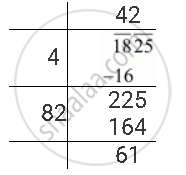Share

# Find the Least Number Which Must Be Added to the Following Number So as to Get a Perfect Square. Also Find the Square Root of the Perfect Square So Obtained 1825 - CBSE Class 8 - Mathematics

ConceptSquare Roots - Finding Square Root by Division Method

#### Question

Find the least number which must be added to the following number so as to get a perfect square. Also find the square root of the perfect square so obtained

1825

#### Solution

The square root of 1825 can be calculated by long division method as follows.The remainder is 61. It represents that the square of 42 is less than 1825.

The next number is 43 and 432 = 1849

Hence, number to be added to 1825 = 432 − 1825 = 1849 − 1825 = 24

The required perfect square is 1849 and  sqrt1849 = 43

Is there an error in this question or solution?

#### APPEARS IN

NCERT Solution for Mathematics Textbook for Class 8 (2018 to Current)
Chapter 6: Squares and Square Roots
Ex. 6.40 | Q: 5.4 | Page no. 108
Solution Find the Least Number Which Must Be Added to the Following Number So as to Get a Perfect Square. Also Find the Square Root of the Perfect Square So Obtained 1825 Concept: Square Roots - Finding Square Root by Division Method.
S